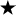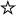home | O'Reilly's CD bookshelfs | FreeBSD | Linux | Cisco | Cisco Exam

#Chapter 2Simple Graphics## 2.2 Point

The Point class encapsulates x and y coordinates within a single object. It is probably one of the most underused classes within Java. Although there are numerous places within AWT where you would expect to see a Point, its appearances are surprisingly rare. Java 1.1 is starting to use Point more heavily. The Point class is most often used when a method needs to return a pair of coordinates; it lets the method return both x and y as a single object. Unfortunately, Point usually is not used when a method requires x and y coordinates as arguments; for example, you would expect the Graphics class to have a version of translate() that takes a point as an argument, but there isn't one.

The Point class does not represent a point on the screen. It is not a visual object; there is no drawPoint() method.

### Point Methods

Variables

The two public variables of Point represent a pair of coordinates. They are accessible directly or use the getLocation() method. There is no predefined origin for the coordinate space.

public int x

The coordinate that represents the horizontal position.

public int y

The coordinate that represents the vertical position.

Constructors

public Point ()

The first constructor creates an instance of Point with an initial x value of 0 and an initial y value of 0.

public Point (int x, int y)

The next constructor creates an instance of Point with an initial x value of x and an initial y value of y.

public Point (Point p)

The last constructor creates an instance of Point from another point, the x value of p.x and an initial y value of p.y.

Locations

public Point getLocation ()The getLocation() method retrieves the current location of this point as a new Point.

public void setLocation (int x, int y)public void move (int x, int y)The setLocation() method changes the point's location to (x, y).

move() is the Java 1.0 name for this method.

public void setLocation (Point p)This setLocation() method changes the point's location to (p.x, p.y).

public void translate (int x, int y)

The translate() method moves the point's location by adding the parameters (x, y) to the corresponding fields of the Point. If the original Point p is (3, 4) and you call p.translate(4, -5), the new value of p is (7, -1).

Miscellaneous methods

public int hashCode ()

The hashCode() method returns a hash code for the point. The system calls this method when a Point is used as the key for a hash table.

public boolean equals (Object object)

The equals() method overrides the Object.equals() method to define equality for points. Two Point objects are equal if their x and y values are equal.

public String toString ()

The toString() method of Point displays the current values of the x and y variables. For example:

```java.awt.Point[x=100,y=200]
```